Author: Tim Cummings

# Factors of a number using python

This exercise is to find all the factors of a given number. Remember that a factor divides evenly into a number without leaving a remainder. We can use the modulo operator (%) to check for any remainder. The program will check all integers from 2 up to but not including the original number. To loop through those numbers we will use the `for` loop combined with the `range()` function.

```number = int(input("Enter the number?"))
for i in range(2,number):
remainder = number % i
quotient = number // i
print(number,"=",i,"x",quotient,"+ remainder",remainder)
if remainder == 0:
print("remainder is zero so",i,"is a factor")
else:
print("remainder is not zero so",i,"is not a factor")
```

Using `print()` in this way shows clearly the flow of the program. However, two lines of code for each potential factor is a lot to read through. To reduce the number of lines of code, `print()` lets us specify a different ending than a new line. If we specify end=’ ‘ then print() will end the line with a space rather than a new line significantly reducing the number of lines of output.

The following program ignores output when the number is not a factor, and displays all the factors on the same line, with a final message whether the original number is prime or composite. Instead of asking for the number to be entered, the program finds all factors for all numbers between 2 and 99 inclusive.

```for number in range(2,100):
print(number,'factors:', end=' ')
prime=True
for i in range(2,number):
if number%i == 0:
print(i, end=' ')
prime=False
if prime:
print('prime')
else:
print('composite')
```
Categories: CoderDojo

# Guessing number game in Python

This exercise is to write a program which asks the user to guess a number and the program will give hints to help the user guess the correct answer.

To display a prompt waiting for a user to enter some data, we use the `input()` function. The `input()` function provides the data as a string, which is a sequence of letters. To convert to an integer number we use the `int()` function.

To control the flow of the program based on the user’s answers, we use `if` and `elif` statements (`elif` is short for “else if”).

```answer=15
guess=None
guess=int(input("Guess a number between 0 and 50 ? "))
print("too high")
print("too low")

### Importing more functionality

Sometimes we want more functionality than is provided in the base python system. In our example we want a random number generator which is available in the `random` module (library). The program becomes a lot more interesting now, because we can’t see the answer by reading the source code.

```import random
guess=None
guess=int(input("Guess a number between 0 and 50 ? "))
print("too high")
print("too low")

In the previous countdown examples, we can put a timer to ensure the countdown happens one second at a time.

```import time
x=10
while x>0:
print(x)
x-=1
time.sleep(1)
print("blast off")
```
Categories: CoderDojo

# Getting started with Python

There are many online resources for beginning in python. Here are a few

https://wiki.python.org/moin/BeginnersGuide/NonProgrammers – Python’s own suggestions for getting started
http://www.letslearnpython.com/learn/ – An online tutorial for kids used at PyCon each year
http://kata.coderdojo.com/wiki/Python_Path – Some international CoderDojo resources

At Kenmore CoderDojo Term 3 2017 we are going to be using Python 3. We will also be using Python with Minecraft. For full setup instructions, refer to https://www.triptera.com.au/wordpress/2017/06/02/coderdojo-minecraft-with-python-setup/

### Python shell

At our first session, we installed Python 3.6.2 and used the IDLE development environment for running python in a shell.

Python can use operators +, -, *, /, //, % and ** to preform mathematical operations

```>>> 5 + 3
8
>>> 5 - 3
2
>>> 5 * 3
15
>>> 5 / 3
1.666666666667
>>> 5 // 3
1
>>> 5 % 3
2
>>> 5 ** 3
125
```

```+ addition - subtraction * multiplication / division (decimal) // division (integer) % modulo (remainder from division) ** to the power of```

### Variables

To store data in memory, create a name for the memory location, and assign it using the `=` sign. These memory locations are called variables because you can change the data in them. Type the variable name by itself to see the value stored in it. You can use variables in mathematical equations.

```>>>speed=50
>>>duration=3
>>>speed
50
>>>speed * duration
150
```

### Python program

When using the shell the commands are not saved. By putting the commands in a file they can be run over and over again without having to be retyped. In IDLE choose `New File` from the `File` menu and type the following

```print(3)
print(2)
print(1)
print("blast off")
```

Save this file from the `File` menu. From the `Run` menu select `Run module F5`. Every time you run it you will see the same output in the shell window.

```3 2 1 blast off```

### Using loops to save typing

Loops are sections of code which are run multiple times. `while` loops will keep looping until a specified condition is `True`. The indented lines indicate which part of the code is included in the loop.

```x = 5
while x > 0:
print(x)
x -= 1
print("blast off")
```

The condition is `x > 0` which is `True` for 10 loops and then becomes `False` when the value of `x` is zero.
The command `x -= 1` is a shorthand for telling python to reduce the value of `x` by 1 every time it is run.
This program gives the following output every time it is run.

```5 4 3 2 1 blast off```

Only one line needs to be changed to start the countdown from a different number.

Categories: CoderDojo

# Python in Minecraft 9 – railway track

To add railway track we need to have some normal rail and some powered rail. Next to every section of powered rail we need to provide a redstone torch.

First we define the items which are not defined in mcpi.block yet

```    RAIL = block.Block(66)
RAIL_POWERED = block.Block(27)
TORCH_REDSTONE = block.Block(76)
```

We are already placing a plain torch every 4 blocks. Now we want to place a redstone torch every 4 blocks but offset by 2. Next to the redstone torch we want two pieces of powered rail, at positions 1 and 2. The rest of the rail is normal rail. This code goes in the second loop where the full tunnel profile is created.

```    if x % 4 == 2:
mc.setBlock(x,y+1,z+1,TORCH_REDSTONE.id,5)
if x % 4 == 1 or x % 4 == 2:
mc.setBlock(x,y+1,z,RAIL_POWERED)
else:
mc.setBlock(x,y+1,z,RAIL)
```

Here is a screenshot showing the railway track in the tunnel.Here is the full code.

```import mcpi.minecraft as minecraft
import mcpi.block as block
mc=minecraft.Minecraft.create()
XMIN = -400 # x value at one end of tunnel.
XMAX = -200 # x value at other end of tunnel. Must be greater than XMIN
GROUND = 72 # y position of tunnel at each end
FLOOR = 10  # minimum value of y at bottom of tunnel
ZPOS = -244 # z position for full length of tunnel
TAIL = 10   # length of horizontal at each end of tunnel

# Define blocks currently missing from mcpi.block
STAIRS_STONE = block.Block(109)
RAIL = block.Block(66)
RAIL_POWERED = block.Block(27)
TORCH_REDSTONE = block.Block(76)

# constants for tunnelvertical function
kx = (XMAX + XMIN) / 2
ky = GROUND + TAIL - (XMAX - XMIN) / 2

# tunnelvertical returns a vertical position of the tunnel
# for each value of the x position (eastposition)
def tunnelvertical(eastposition):
y = abs(eastposition - kx) + ky
if y < FLOOR:
return FLOOR
if y > GROUND:
return GROUND
return y

# set the z coordinate for the tunnel which doesn't change for the full length
z = ZPOS

# Initial loop to create a route made of solid glass with a stone base
for x in range(XMIN,XMAX+1):
y = tunnelvertical(x)
mc.setBlocks(x,y,z-2,x,y+6,z+2,block.GLASS)
mc.setBlocks(x,y,z-1,x,y,z+1,block.STONE)

# Initialise previous value of y so can determine if stairs going up or down
yprev = tunnelvertical(XMIN)
# Second loop to convert solid glass into a tunnel
for x in range(XMIN+1,XMAX):
y = tunnelvertical(x)
# replace centre glass with air to make it a tunnel
mc.setBlocks(x,y+1,z-1,x,y+5,z+1,block.AIR)
# place a torch every 4 positions to light the tunnel
# variation 5 = torch facing up
# every 4 blocks
# pos 0: rail and normal torch
# pos 1: powered rail
# pos 2: powered rail and redstone torch
# pos 3: rail
if x % 4 == 0:
mc.setBlock(x,y+1,z+1,block.TORCH.id,5)
if x % 4 == 2:
mc.setBlock(x,y+1,z+1,TORCH_REDSTONE.id,5)
if x % 4 == 1 or x % 4 == 2:
mc.setBlock(x,y+1,z,RAIL_POWERED)
else:
mc.setBlock(x,y+1,z,RAIL)
# check if stairs are going up or down
if y > yprev:
# variation 0 = stairs ascending to the east
mc.setBlock(x,y,z-1,STAIRS_STONE.id,0)
if y < yprev:
# variation 1 = stairs ascending to the west
mc.setBlock(x-1,yprev,z-1,STAIRS_STONE.id,1)
yprev=y
```
Categories: CoderDojo

# Python in Minecraft 8 – stairs

To add stairs we need to know if the tunnel is descending or ascending to the east. We do this by creating a variable called yprev which stores the previous vertical position of the tunnel to the current position. We are creating the tunnel by increasing the value of x which means we are heading east. Hence if the current vertical position is more than the previous vertical position, the stairs are ascending to the east. If the current vertical position is less than the previous vertical position, the stairs are ascending to the west (descending to the east). If they are the same then we are not ascending or descending so no stairs are needed. At the end of the loop we need to store the current value of y in yprev so next time through the loop we will know the previous value. Check the full code to see where we initialise yprev before the loop.

```    # check if stairs are going up or down
if y > yprev:
# variation 0 = stairs ascending to the east
mc.setBlock(x,y,z-1,STAIRS_STONE.id,0)
if y < yprev:
# variation 1 = stairs ascending to the west
mc.setBlock(x-1,yprev,z-1,STAIRS_STONE.id,1)
yprev=y
```

We have decided to use stone stairs which are not predefined in Block.py in the modded mcpi. Hence we define it manually here.

```    STAIRS_STONE = block.Block(109)
```

Here is a screenshot showing the ascending stairs at the end of the tunnel.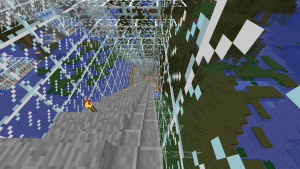Here is the full code.

```import mcpi.minecraft as minecraft
import mcpi.block as block
mc=minecraft.Minecraft.create()
XMIN = -400 # x value at one end of tunnel.
XMAX = -200 # x value at other end of tunnel. Must be greater than XMIN
GROUND = 72 # y position of tunnel at each end
FLOOR = 10  # minimum value of y at bottom of tunnel
ZPOS = -244 # z position for full length of tunnel
TAIL = 10   # length of horizontal at each end of tunnel

# Define blocks currently missing from mcpi.block
STAIRS_STONE = block.Block(109)

# constants for tunnelvertical function
kx = (XMAX + XMIN) / 2
ky = GROUND + TAIL - (XMAX - XMIN) / 2

# tunnelvertical returns a vertical position of the tunnel
# for each value of the x position (eastposition)
def tunnelvertical(eastposition):
y = abs(eastposition - kx) + ky
if y < FLOOR:
return FLOOR
if y > GROUND:
return GROUND
return y

# set the z coordinate for the tunnel which doesn't change for the full length
z = ZPOS

# Initial loop to create a route made of solid glass with a stone base
for x in range(XMIN,XMAX+1):
y = tunnelvertical(x)
mc.setBlocks(x,y,z-2,x,y+6,z+2,block.GLASS)
mc.setBlocks(x,y,z-1,x,y,z+1,block.STONE)

# Initialise previous value of y so can determine if stairs going up or down
yprev = tunnelvertical(XMIN)
# Second loop to convert solid glass into a tunnel
for x in range(XMIN+1,XMAX):
y = tunnelvertical(x)
# replace centre glass with air to make it a tunnel
mc.setBlocks(x,y+1,z-1,x,y+5,z+1,block.AIR)
# place a torch every 4 positions to light the tunnel
# variation 5 = torch facing up
if x % 4 == 0:
mc.setBlock(x,y+1,z+1,block.TORCH.id,5)
# check if stairs are going up or down
if y > yprev:
# variation 0 = stairs ascending to the east
mc.setBlock(x,y,z-1,STAIRS_STONE.id,0)
if y < yprev:
# variation 1 = stairs ascending to the west
mc.setBlock(x-1,yprev,z-1,STAIRS_STONE.id,1)
yprev=y
```
Categories: CoderDojo

# Python in Minecraft 7 – define tunnel route in function

In the previous example the tunnel was horizontal so the value of y for the tunnel was always constant. To make the tunnel route more complicated without having duplicate code we define a function called tunnelvertical(eastposition). For any value of the east position (x value) this function will return the vertical position of the tunnel.

```def tunnelvertical(eastposition):
y = abs(100 - (eastposition - XMIN))
if y < 10:
return 10
if y > 72:
return 72
return y
```

XMIN is a constant we have previously defined in the code showing where the tunnel starts. The shape of this tunnel is horizontal at ground level, then slopes down until it reaches level 10, goes horizontal for a while, then slopes up until it reaches ground level again and finishes off horizontal.

Here is the full code

```import mcpi.minecraft as minecraft
import mcpi.block as block
mc=minecraft.Minecraft.create()
XMIN = -400 # x value at one end of tunnel.
XMAX = -200 # x value at other end of tunnel. Must be greater than XMIN
GROUND = 72 # y position of tunnel at each end
FLOOR = 10  # minimum value of y at bottom of tunnel
ZPOS = -222 # z position for full length of tunnel
TAIL = 10   # length of horizontal at each end of tunnel

# constants for tunnelvertical function
kx = (XMAX + XMIN) / 2
ky = GROUND + TAIL - (XMAX - XMIN) / 2

# tunnelvertical returns a vertical position of the tunnel
# for each value of the x position (eastposition)
def tunnelvertical(eastposition):
y = abs(eastposition - kx) + ky
if y < FLOOR:
return FLOOR
if y > GROUND:
return GROUND
return y

# set the z coordinate for the tunnel which doesn't change for the full length
z = ZPOS

# Initial loop to create a route made of solid glass with a stone base
for x in range(XMIN,XMAX+1):
y = tunnelvertical(x)
mc.setBlocks(x,y,z-2,x,y+6,z+2,block.GLASS)
mc.setBlocks(x,y,z-1,x,y,z+1,block.STONE)

# Second loop to convert solid glass into a tunnel
for x in range(XMIN+1,XMAX):
y = tunnelvertical(x)
# replace centre glass with air to make it a tunnel
mc.setBlocks(x,y+1,z-1,x,y+5,z+1,block.AIR)
# place a torch every 4 positions to light the tunnel
if x % 4 == 0:
mc.setBlock(x,y+1,z+1,block.TORCH.id,5)
```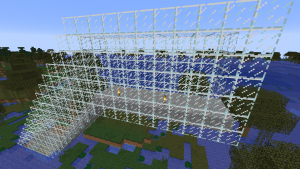Categories: CoderDojo

# Python in Minecraft 6 – stone floor tunnel with torches

In the previous example the tunnel was glass all around. To make a more interesting tunnel this example adds a stone floor and adds a torch. We will develop this tunnel in two stages. The first stage is to create a profile showing what a cross-section of the tunnel will look like for any value of x.

`nano tunnelprofile2.py`

```import mcpi.minecraft as minecraft
import mcpi.block as block
mc=minecraft.Minecraft.create()
x = -190
y = 72
z = -222
mc.setBlocks(x,y,z-2,x,y+6,z+2,block.GLASS)
mc.setBlocks(x,y,z-1,x,y,z+1,block.STONE)
mc.setBlocks(x,y+1,z-1,x,y+5,z+1,block.AIR)
mc.setBlock(x,y+1,z+1,block.TORCH.id,5)```

`python3 tunnelprofile2.py`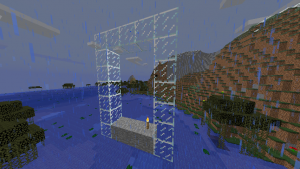The second stage is to create a loop to draw each cross section of the tunnel at each value of x between xmin and xmax. Note that at the ends we ant the wall of glass to stay so that lava and water don’t flow into the tunnel should it get built next to these liquids. We have achieved this with two loops. The first loop goes the full length of the tunnel from xmin to xmax. The second loop which adds air and torches starts and ends one position in from the ends. Torches are added every 4 positions by using the modulo (%) operator.

`nano stonefloortunnel.py`

```import mcpi.minecraft as minecraft
import mcpi.block as block
mc=minecraft.Minecraft.create()
xmin = -224
xmax = -200
y = 72
z = -222
for x in range(xmin,xmax+1):
mc.setBlocks(x,y,z-2,x,y+6,z+2,block.GLASS)
mc.setBlocks(x,y,z-1,x,y,z+1,block.STONE)
for x in range(xmin+1,xmax):
mc.setBlocks(x,y+1,z-1,x,y+5,z+1,block.AIR)
if x % 4 == 0:
mc.setBlock(x,y+1,z+1,block.TORCH.id,5)```

`python3 stonefloortunnel.py`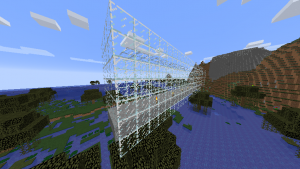Categories: CoderDojo

# Python in Minecraft 5 – hollow tunnel

## Creating a hollow tunnel with walls at each end

To create an air space in the tunnel we can use another loop. However, the second loop should not go all the way to the ends because we want walls at each end. This is to stop water and lava flowing into our tunnel should the ends of the tunnel meet these liquids.

`nano hollowglasstunnel.py`

```import mcpi.minecraft as minecraft
import mcpi.block as block
mc=minecraft.Minecraft.create()
xmin = -224
xmax = -200
y = 72
z = -222
for x in range(xmin,xmax+1):
mc.setBlocks(x,y,z-2,x,y+6,z+2,block.GLASS)
for x in range(xmin+1,xmax):
mc.setBlocks(x,y+1,z-1,x,y+5,z+1,block.AIR)
```

`python3 hollowglasstunnel.py`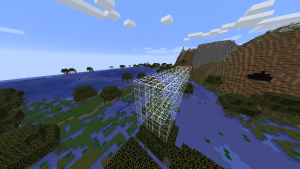Categories: CoderDojo

# Python in Minecraft 4 – Creating a solid tunnel

Our next exercise it to create a tunnel of glass filled with air. Glass is useful because it is transparent. To start with the tunnel will be horizontal heading east which means the x coordinate will be increasing and the y and z coordinates will keep constant values. We could use the setBlocks command for the entire length of the tunnel. However we want to keep the code flexible enough that later on the tunnel could start ascending or descending, i.e. the y coordinate could start increasing or decreasing. The profile of the tunnel which will be a wall of glass 5 blocks across and 7 blocks high. Here is the code to create a profile of the tunnel for one value of the x coordinate.

`nano tunnelprofile.py`

```import mcpi.minecraft as minecraft
import mcpi.block as block
mc=minecraft.Minecraft.create()
x = -200
y = 72
z = -222
mc.setBlocks(x,y,z-2,x,y+6,z+2,block.GLASS)
```

`python3 tunnelprofile.py`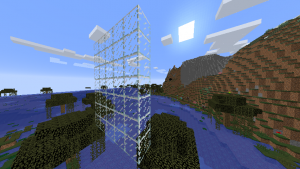A loop can be added which will draw the profile of the tunnel over and over again for a range of different x values. Note how important indentation is in python. I am indenting 4 spaces when in a loop.

`nano solidglasstunnel.py`

```import mcpi.minecraft as minecraft
import mcpi.block as block
mc=minecraft.Minecraft.create()
xmin = -224
xmax = -200
y = 72
z = -222
for x in range(xmin,xmax+1):
mc.setBlocks(x,y,z-2,x,y+6,z+2,block.GLASS)
```

`python3 solidglasstunnel.py`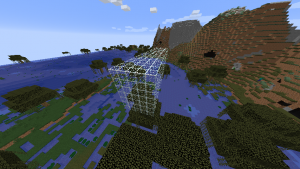Categories: CoderDojo

# Python in Minecraft 3 – Placing many blocks at once

The previous example showed that python scripts could quickly become very long if each block had to be set individually. Fortunately there is the `setBlocks` command which can set many blocks at once. It also has two forms.

```mc.setBlocks(x1,y1,z1,x2,y2,z2,block) mc.setBlocks(x1,y1,z1,x2,y2,z2,block.id,variation)```

The minecraft coordinates (x1,y1,z1) represent one corner of the rectangular prism about to be set. The minecraft coordinates (x2,y2,z2) represent the diagonally opposite corner. The previous example can be rewritten to use the `setBlocks` command. The glass is set first otherwise it would overwrite the setting of the middle block. In this example the middle block is air which is equivalent to there being no block.

`nano placeglassblocks.py`

```import mcpi.minecraft as minecraft
import mcpi.block as block
mc=minecraft.Minecraft.create()
mc.setBlocks(-220,72,-223,-218,74,-221,block.GLASS)
mc.setBlock(-219,73,-222, block.AIR)
```

`python3 placeglassblocks.py`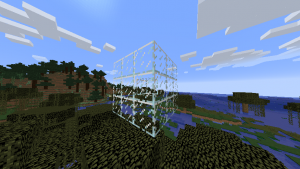Categories: CoderDojo